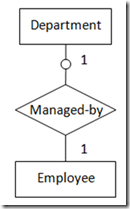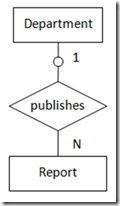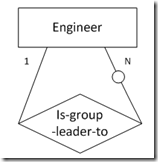## 数据库设计Step by Step (9)——ER-to-SQL转化 转

1. 转化得到的SQL表与原始实体包含相同信息内容。该类转化一般适用于：

2. 转化得到的SQL表除了包含原始实体的信息内容之外，还包含原始实体父实体的外键。该类转化一般适用于：

3. 由“关系”转化得到的SQL表，该表包含“关系”所涉及的所有实体的外键，以及该“关系”自身的属性信息。该类转化一般适用于：

1. 当实体之间的关系是可选的，SQL表中的外键列允许为NULL。

2. 当实体之间的关系是强制的，SQL表中的外键列不允许为NULL。

3. 由“多对多”关系转化得到的SQL表，其中的任意外键列都不允许为NULL。

1. “一对一”，两实体都为强制存在

 1 2 3 4 5 6 7 8 9 10 11 12 13 14 `create`  `table`  `report` `(` `    ` `report_no ` `integer` `,` `    ` `report_name ` `varchar` `(256),` `    ` `primary`  `key` `(report_no)` `);` `create`  `table`  `abbreviation` `(` `    ` `abbr_no ` `char` `(6),` `    ` `report_no ` `integer`  `not`  `null`  `unique` `,` `    ` `primary`  `key` `(abbr_no),` `    ` `foreign`  `key` `(report_no) ` `references`  `report` `        ` `on`  `delete`  `cascade`  `on`  `update`  `cascade` `);`

2. “一对一”，一实体可选存在，另一实体强制存在（图2  “一对一”，一实体可选存在，另一实体强制存在）

 1 2 3 4 5 6 7 8 9 10 11 12 13 14 15 `create`  `table`  `employee` `(` `    ` `emp_id ` `char` `(10),` `    ` `emp_name ` `char` `(20),` `    ` `primary`  `key` `(emp_id)` `);` `create`  `table`  `department` `(` `    ` `dept_no ` `integer` `,` `    ` `dept_name ` `char` `(20),` `    ` `mgr_id ` `char` `(10)` `not`  `null`  `unique` `,` `    ` `primary`  `key` `(dept_no),` `    ` `foreign`  `key` `(mgr_id)` `references`  `employee` `        ` `on`  `update`  `cascade` `);`

3. “一对一”，两实体都为可选存在

 1 2 3 4 5 6 7 8 9 10 11 12 13 14 `create`  `table`  `engineer` `(` `    ` `emp_id ` `char` `(10),` `    ` `emp_name ` `char` `(20),` `    ` `primary`  `key` `(emp_id)` `);` `create`  `table`  `desktop` `(` `    ` `desktop_no ` `integer` `,` `    ` `emp_id ` `char` `(10),` `    ` `primary`  `key` `(desktop_no),` `    ` `foreign`  `key` `(emp_id)` `references`  `engineer` `        ` `on`  `delete`  `set`  `null`  `on`  `update`  `cascade` `);`

4. “一对多”，两实体都为强制存在

 1 2 3 4 5 6 7 8 9 10 11 12 13 14 15 `create`  `table`  `department` `(` `    ` `dept_no ` `integer` `,` `    ` `dept_name ` `char` `(20),` `    ` `primary`  `key` `(dept_no)` `);` `create`  `table`  `employee` `(` `    ` `emp_id ` `char` `(10),` `    ` `emp_name ` `char` `(20),` `    ` `dept_no ` `integer`  `not`  `null` `,` `    ` `primary`  `key` `(emp_id),` `    ` `foreign`  `key` `(dept_no)` `references`  `department` `        ` `on`  `update`  `cascade` `);`

5. “一对多”，一实体可选存在，另一实体强制存在（图5  “一对多”，一实体可选存在，另一实体强制存在）

 1 2 3 4 5 6 7 8 9 10 11 12 13 14 `create`  `table`  `department` `(` `    ` `dept_no ` `integer` `,` `    ` `dept_name ` `char` `(20),` `    ` `primary`  `key` `(dept_no)` `);` `create`  `table`  `report` `(` `    ` `report_no ` `integer` `,` `    ` `dept_no ` `integer` `,` `    ` `primary`  `key` `(report_no),` `    ` `foreign`  `key` `(dept_no)` `references`  `department` `        ` `on`  `delete`  `set`  `null`  `on`  `update`  `cascade` `);`

6. “多对多”，两实体都为可选存在

 1 2 3 4 5 6 7 8 9 10 11 12 13 14 15 16 17 18 19 20 `create`  `table`  `engineer` `(` `    ` `emp_id ` `char` `(10),` `    ` `primary`  `key` `(emp_id)` `);` `create`  `table`  `prof_assoc` `(` `    ` `assoc_name ` `varchar` `(256),` `    ` `primary`  `key` `(assoc_name)` `);` `create`  `table`  `belongs_to` `(` `    ` `emp_id ` `char` `(10),` `    ` `assoc_name ` `varchar` `(256),` `    ` `primary`  `key` `(emp_id, assoc_name),` `    ` `foreign`  `key` `(emp_id) ` `references`  `engineer` `        ` `on`  `delete`  `cascade`  `on`  `update`  `cascade` `,` `    ` `foreign`  `key` `(assoc_name)` `references`  `prof_assoc` `        ` `on`  `delete`  `cascade`  `on`  `update`  `cascade` `);`

7. “一对一”，两实体都为可选存在

 1 2 3 4 5 6 7 8 `create`  `table`  `employee` `(` `    ` `emp_id ` `char` `(10),` `    ` `emp_name ` `char` `(20),` `    ` `spouse_id ` `char` `(10),` `    ` `primary`  `key` `(emp_id),` `    ` `foreign`  `key` `(spouse_id) ` `references`  `employee` `);`

8. “一对多”，“一”端为强制存在，“多”端为可选存在（图8  “一对多”，“一”端为强制存在，“多”端为可选存在）

 1 2 3 4 5 6 7 `create`  `table`  `engineer` `(` `    ` `emp_id ` `char` `(10),` `    ` `leader_id ` `char` `(10) ` `not`  `null` `,` `    ` `primary`  `key` `(emp_id),` `    ` `foreign`  `key` `(leader_id)` `references`  `engineer` `);`

“多对多”回归关系无论是可选存在的还是强制存在的都需新增一张关系表，表中的外键列须为NOT  NULL。

9. “多对多”，两端都为可选存在

 1 2 3 4 5 6 7 8 9 10 11 12 13 14 15 `create`  `table`  `person` `(` `    ` `person_id ` `char` `(10),` `    ` `person_name ` `char` `(20),` `    ` `primary`  `key` `(person_id)` `);` `create`  `table`  `friend` `(` `    ` `person_id ` `char` `(10),` `    ` `friend_id ` `char` `(10),` `    ` `primary`  `key` `(person_id, friend_id),` `    ` `foreign`  `key` `(person_id) ` `references`  `person,` `    ` `foreign`  `key` `(friend_id) ` `references`  `person,` `    ` `check` `(person_id < friend_id)` `);`

10. “一对一对一”三元关系

1名技术员在1个项目中使用特定的1本记事簿

1本记事簿在1个项目中只属于1名技术员

1名技术员的1本记事簿只用于记录1个项目

 1 2 3 4 5 6 7 8 9 10 11 12 13 14 15 16 17 18 19 20 21 22 23 24 25 26 27 28 29 30 `create`  `table`  `technician` `(` `    ` `emp_id ` `char` `(10),` `    ` `primary`  `key` `(emp_id)` `);` `create`  `table`  `project` `(` `    ` `project_name ` `char` `(20),` `    ` `primary`  `key` `(project_name)` `);` `create`  `table`  `notebook` `(` `    ` `notebook_no ` `integer` `,` `    ` `primary`  `key` `(notebook_no)` `);` `create`  `table`  `uses_notebook` `(` `    ` `emp_id ` `char` `(10),` `    ` `project_name ` `char` `(20),` `    ` `notebook_no ` `integer`  `not`  `null` `,` `    ` `primary`  `key` `(emp_id, project_name),` `    ` `foreign`  `key` `(emp_id)` `references`  `technician` `        ` `on`  `delete`  `cascade`  `on`  `update`  `cascade` `,` `    ` `foreign`  `key` `(project_name)` `references`  `project` `        ` `on`  `delete`  `cascade`  `on`  `update`  `cascade` `,` `    ` `foreign`  `key` `(notebook_no)` `references`  `notebook` `        ` `on`  `delete`  `cascade`  `on`  `update`  `cascade` `,` `    ` `unique` `(emp_id, notebook_no),` `    ` `unique` `(project_name, notebook_no)` `);`

emp_id, project_name -> notebook_no

emp_id, notebook_no -> project_name

project_name, notebook_no -> emp_id

11. “一对一对多”三元关系

1名员工在1个地点只能做1个项目

1个地点的1个项目可能有多名员工参与

 1 2 3 4 5 6 7 8 9 10 11 12 13 14 15 16 17 18 19 20 21 22 23 24 25 26 27 28 29 30 `create`  `table`  `employee` `(` `    ` `emp_id ` `char` `(10),` `    ` `emp_name ` `char` `(20),` `    ` `primary`  `key` `(emp_id)` `);` `create`  `table`  `project` `(` `    ` `project_name ` `char` `(20),` `    ` `primary`  `key` `(project_name)` `);` `create`  `table`  `location` `(` `    ` `loc_name ` `char` `(15),` `    ` `primary`  `key` `(loc_name)` `);` `create`  `table`  `assigned_to` `(` `    ` `emp_id ` `char` `(10),` `    ` `project_name ` `char` `(20),` `    ` `loc_name ` `char` `(15) ` `not`  `null` `,` `    ` `primary`  `key` `(emp_id, project_name),` `    ` `foreign`  `key` `(emp_id)` `references`  `employee` `        ` `on`  `delete`  `cascade`  `on`  `update`  `cascade` `,` `    ` `foreign`  `key` `(project_name)` `references`  `project` `        ` `on`  `delete`  `cascade`  `on`  `update`  `cascade` `,` `    ` `foreign`  `key` `(loc_name)` `references`  `location` `        ` `on`  `delete`  `cascade`  `on`  `update`  `cascade` `,` `    ` `unique` `(emp_id, loc_name)` `);`

emp_id, loc_name -> project_name

emp_id, project_name -> loc_name

12. “一对多对多”三元关系

1个项目中的1名工程师只会有1名经理

1个项目中的1名经理会带领多名工程师做该项目

1名经理和他手下的1名工程师可能参与多个项目

 1 2 3 4 5 6 7 8 9 10 11 12 13 14 15 16 17 18 19 20 21 22 23 24 25 26 27 28 `create`  `table`  `project` `(` `    ` `project_name ` `char` `(20),` `    ` `primary`  `key` `(project_name)` `);` `create`  `table`  `manager` `(` `    ` `mgr_id ` `char` `(10),` `    ` `primary`  `key` `(mgr_id)` `);` `create`  `table`  `engineer` `(` `    ` `emp_id ` `char` `(10),` `    ` `primary`  `key` `(emp_id)` `);` `create`  `table`  `manages` `(` `    ` `project_name ` `char` `(20),` `    ` `mgr_id ` `char` `(10) ` `not`  `null` `,` `    ` `emp_id ` `char` `(10),` `    ` `primary`  `key` `(project_name, emp_id),` `    ` `foreign`  `key` `(project_name)` `references`  `project` `        ` `on`  `delete`  `cascade`  `on`  `update`  `cascade` `,` `    ` `foreign`  `key` `(mgr_id)` `references`  `manager` `        ` `on`  `delete`  `cascade`  `on`  `update`  `cascade` `,` `    ` `foreign`  `key` `(emp_id)` `references`  `engineer` `        ` `on`  `delete`  `cascade`  `on`  `update`  `cascade` `);`

project_name, emp_id -> mgr_id

13. “多对多对多”三元关系

1名员工在1个项目中可以运用多种技能

1名员工的1项技能可以在多个项目中运用

1个项目中的1项技能可以被参与该项目的多名员工运用

 1 2 3 4 5 6 7 8 9 10 11 12 13 14 15 16 17 18 19 20 21 22 23 24 25 26 27 28 29 `create`  `table`  `employee` `(` `    ` `emp_id ` `char` `(10),` `    ` `emp_name ` `char` `(20),` `    ` `primary`  `key` `(emp_id)` `);` `create`  `table`  `skill` `(` `    ` `skill_type ` `char` `(15),` `    ` `primary`  `key` `(skill_type)` `);` `create`  `table`  `project` `(` `    ` `project_name ` `char` `(20),` `    ` `primary`  `key` `(project_name)` `);` `create`  `table`  `sill_used` `(` `    ` `emp_id ` `char` `(10),` `    ` `skill_type ` `char` `(15),` `    ` `project_name ` `char` `(20),` `    ` `primary`  `key` `(emp_id, skill_type, project_name),` `    ` `foreign`  `key` `(emp_id)` `references`  `employee` `        ` `on`  `delete`  `cascade`  `on`  `update`  `cascade` `,` `    ` `foreign`  `key` `(skill_type)` `references`  `skill` `        ` `on`  `delete`  `cascade`  `on`  `update`  `cascade` `,` `    ` `foreign`  `key` `(project_name)` `references`  `project` `        ` `on`  `delete`  `cascade`  `on`  `update`  `cascade` `);`

14. 泛化层次关系

 1 2 3 4 5 6 7 8 9 10 11 12 13 14 15 16 17 18 19 20 21 22 23 `create`  `table`  `individual` `(` `    ` `indiv_id ` `char` `(10),` `    ` `indiv_name ` `char` `(20),` `    ` `indiv_addr ` `char` `(20),` `    ` `primary`  `key` `(indiv_id)` `);` `create`  `table`  `employee` `(` `    ` `emp_id ` `char` `(10),` `    ` `job_title ` `char` `(15),` `    ` `primary`  `key` `(emp_id),` `    ` `foreign`  `key` `(emp_id)` `references`  `individual` `        ` `on`  `delete`  `cascade`  `on`  `update`  `cascade` `);` `create`  `table`  `customer` `(` `    ` `cust_no ` `char` `(10),` `    ` `cust_credit ` `char` `(12),` `    ` `primary`  `key` `(cust_no),` `    ` `foreign`  `key` `(cust_no)` `references`  `individual` `        ` `on`  `delete`  `cascade`  `on`  `update`  `cascade` `);`

1. 把每一个实体转化为一张表，其中包含键和非键属性。

2. 把每一个“多对多”二元或二元回归关系转化为一张表，其中包含实体的键和关系的属性。

3. 把三元及更高元（n元）关系转化为一张表。

“多对多”二元关系转化

ER-to-SQL转化步骤示例

1. 直接由实体生成的SQL表有：

Division          Department          Employee          Manager          Secretary          Engineer

Technician     Skill                          Project                Location           Prof_assoc       Desktop

Workstation

2. 由“多对多”二元关系及“多对多”二元回归关系生成的SQL表有：

belongs_to

3. 由三元关系生成的SQL表有：

skill_used     assigned_to

1. 通过一些简单的规则就能把ER模型中的实体、属性和关系转化为SQL表。

2. 实体在转化为表的过程中，其中的属性一一被映射为表的属性。

3. “一对一”或“一对多”关系中的“子”端实体转化成的SQL表必须包含另一端实体的主键，作为外键。

4. “多对多”关系转化为一张表，包含相关实体的主键，复合组成其自身的主键。同时这些键在SQL中定义为外键分别指向各自的实体。

5. 三元或n元关系被转化为一张表，包含相关实体的主键。这些键在SQL中定义为外键。这些键中的子集定义为主键，其基于该关系的函数依赖。

6. 泛化层次的转化规则要求子类实体从超类实体继承主键。

7. ER图中的可选约束在转化为SQL时，表现为关系的某一端实体允许为null。在ER图中没有明确标识可选约束时，创建表时默认not null约束。### 自由的角马

m18633778874
2017/12/08
0
0
Steps to configure Oracle 11g Data Guard Physical Standby – Active Data Guard Part-I

Steps to configure Oracle 11g Data Guard Physical Standby – Active Data Guard Part-I Published 3 years ago by Jignesh Jethwa Here in this article I am going to cover steps by s......

rootliu
2017/10/28
3K
0
12c：CREATE DATABASE——DBCA

ryan_bai
2018/01/04
0
0

qq_35246620
2017/11/18
0
0
SQLite之C++增删改查（详解）

╰☆╮絠ㄚòひ才倖❤福`
2012/08/23
5.4K
1

JS基础-该如何理解原型、原型链？

JS的原型、原型链一直是比较难理解的内容，不少初学者甚至有一定经验的老鸟都不一定能完全说清楚，更多的"很可能"是一知半解，而这部分内容又是JS的核心内容，想要技术进阶的话肯定不能对这个...

OBKoro1

7
0

14
0
OSChina 周一乱弹 —— 熟悉的味道，难道这就是恋爱的感觉

Osc乱弹歌单（2019）请戳（这里） 【今日歌曲】 @xiaoshiyue ：好久没分享歌了分享张碧晨的单曲《今后我与自己流浪》 《今后我与自己流浪》- 张碧晨 手机党少年们想听歌，请使劲儿戳（这里）...

3K
24
SpringBoot中 集成 redisTemplate 对 Redis 的操作（二）

SpringBoot中 集成 redisTemplate 对 Redis 的操作（二） List 类型的操作 1、 向列表左侧添加数据 Long leftPush = redisTemplate.opsForList().leftPush("name", name); 2、 向列表右......

TcWong

46
0

FAT_mt

6
0